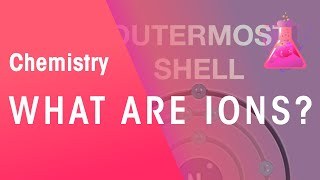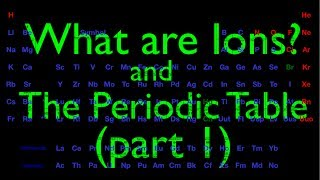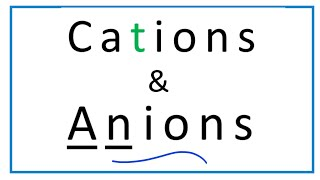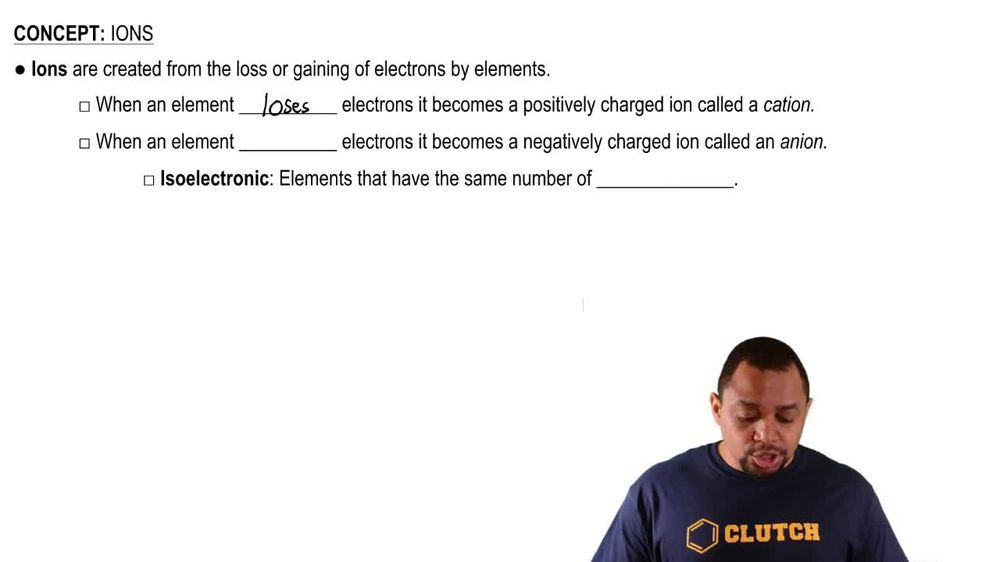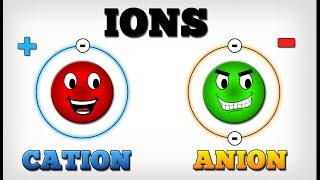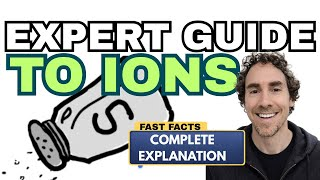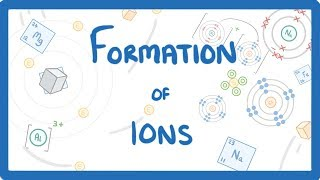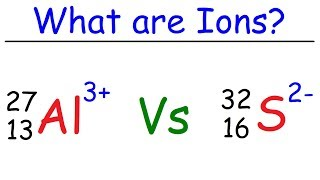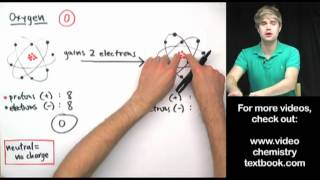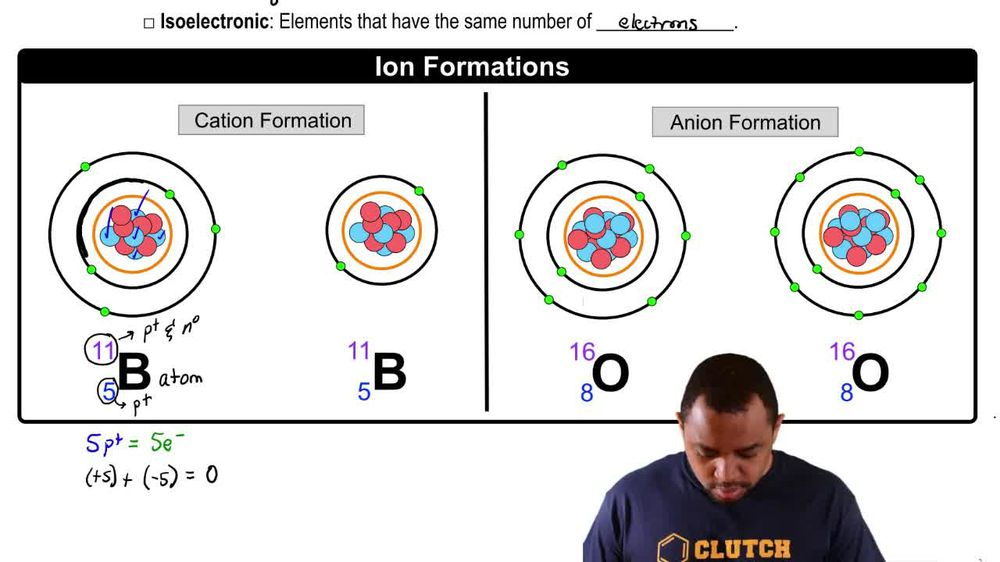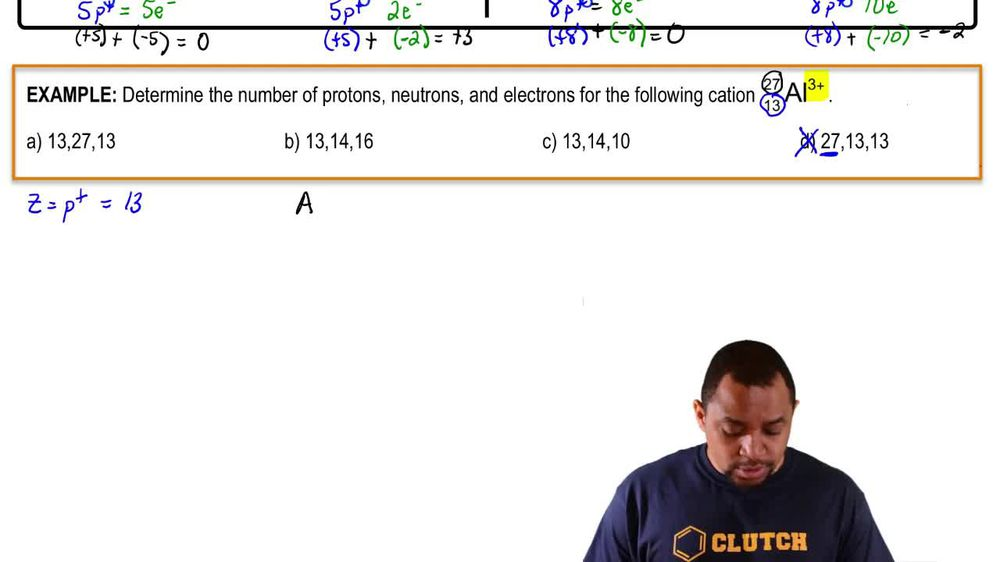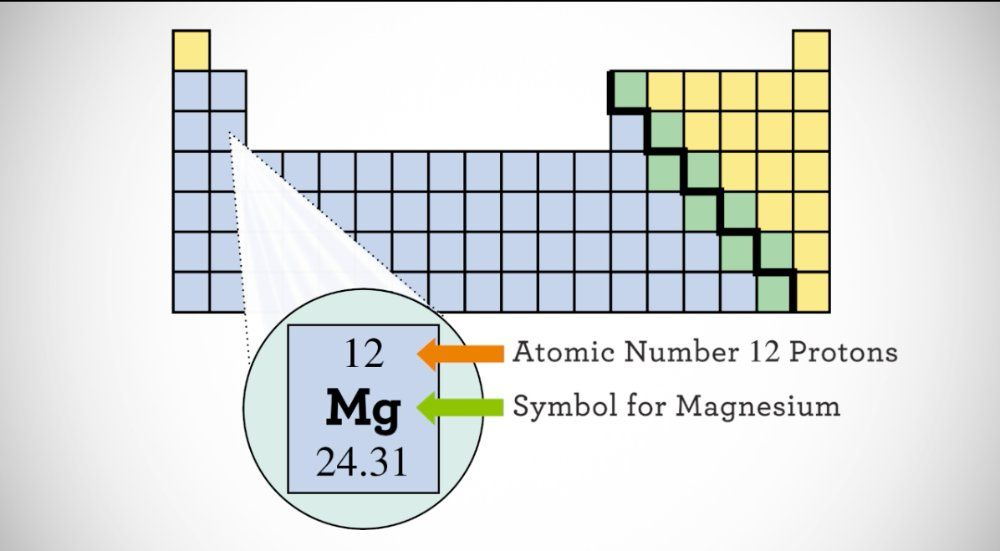Start typing, then use the up and down arrows to select an option from the list.
1. 2. Atoms & Elements2. Ions
Problem

# How many protons and electrons are in each of the following ions? (c) Se2-

Relevant Solution1m
Play a video:
Hey everyone in this example, we need to figure out how many protons and electrons are in an an ion of Aston teen. We want to recall that our chemical symbol is going to have a A in the left hand superscript for atomic mass and a Z in the left hand subscript for our atomic number. We want to recall that Z. The atomic number is going to equal our number of protons. And then in neutral atoms only it equals also our number of electrons. So when we find A 17 on the periodic table, We see that it's in group seven a and it has a atomic number equal to 85. And so therefore we would say that we have 85 protons In our a 17. Now for our ion of a 17, we want to recall that a negative charge means that we gain electrons. So we're going to take Those 85 protons. And in a neutral atom that would equal 85 electrons. Sorry, 85 electrons in a neutral atom. But because we have a charged atom here with a negative charge, we would take 85 and go ahead and add one electron to give us a total of 86 electrons. And so to complete this example, the only correct choice is choice a which states that we have 85 protons and 86 electrons in our an ion a 17. If you have any questions, leave them down below and I will see everyone in the next practice video